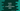# Kotlin program to print a multiplication table## Kotlin program to print a multiplication table:

In this post, we will learn how to print a multiplication table in Kotlin. The program will take one number as input from the user and print the multiplication table i.e. the table of multiplication from 1 to that number.

### Kotlin program:

Below is the complete kotlin program:

``````package com.company

fun main() {
println("Enter a number : ")

for (i in 1..10) {
println("\$givenNumber * \$i = " + (givenNumber * i))
}
}``````

In this program,

• we are reading a number as input from the user and storing it in givenNumber
• Using a for loop, we are printing the multiplication table.
• Inside the loop, it is printing the multiplication table.

It will print output as like below:

``````Enter a number :
4
4 * 1 = 4
4 * 2 = 8
4 * 3 = 12
4 * 4 = 16
4 * 5 = 20
4 * 6 = 24
4 * 7 = 28
4 * 8 = 32
4 * 9 = 36
4 * 10 = 40``````